Get inspired by the success stories of our students in IIT JAM MS, ISI  MStat, CMI MSc DS.  Learn More

# ISI MStat PSB 2009 Problem 1 | Nilpotent MatricesThis is a very simple sample problem from ISI MStat PSB 2009 Problem 1. It is based on basic properties of Nilpotent Matrices and Skew-symmetric Matrices. Try it !

## Problem- ISI MStat PSB 2009 Problem 1

(a) Let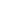be an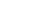matrix such that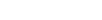where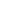denotes the identity matrix. Show thatis non-singular.

(b) Give an example of a non-zero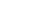real matrixsuch that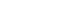for all real vectors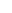.

### Prerequisites

Nilpotent Matrix

Eigenvalues

Skew-symmetric Matrix

## Solution :

The first part of the problem is quite easy,

It is given that for amatrix, we have, so,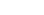is a nilpotet matrix, right !

And we know that all the eigenvalues of a nilpotent matrix are. Hence all the eigenvalues ofare 0.

Now let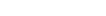be the eigenvalues of the matrix. So, the eigenvalues of the nilpotent matrixare of form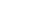where,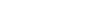. Now since,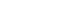which implies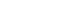, for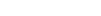.

Since all the eigenvalues ofare non-zero, infact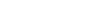. Hence our required propositon.

(b) Now this one is quite interesting,

If for anymatrix, the Quadratic form of that matrix with respect to a vector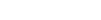is of form,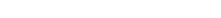where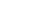and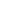are the elements of the matrix. Now if we equate that with, what condition should it impose on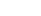and!! I leave it as an exercise for you to complete it. Also Try to generalize it you will end up with a nice result.

## Food For Thought

Now, extending the first part of the question,is invertible right !! So, can you prove that we can always get two vectors from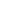, sayand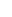, such that the necessary and sufficient condition for the invertiblity of the matrix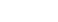is "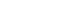must be different from" !!

This is a very important result for Statistics Students !! Keep thinking !!

## Subscribe to Cheenta at Youtube

This is a very simple sample problem from ISI MStat PSB 2009 Problem 1. It is based on basic properties of Nilpotent Matrices and Skew-symmetric Matrices. Try it !

## Problem- ISI MStat PSB 2009 Problem 1

(a) Letbe anmatrix such thatwheredenotes the identity matrix. Show thatis non-singular.

(b) Give an example of a non-zeroreal matrixsuch thatfor all real vectors.

### Prerequisites

Nilpotent Matrix

Eigenvalues

Skew-symmetric Matrix

## Solution :

The first part of the problem is quite easy,

It is given that for amatrix, we have, so,is a nilpotet matrix, right !

And we know that all the eigenvalues of a nilpotent matrix are. Hence all the eigenvalues ofare 0.

Now letbe the eigenvalues of the matrix. So, the eigenvalues of the nilpotent matrixare of formwhere,. Now since,which implies, for.

Since all the eigenvalues ofare non-zero, infact. Hence our required propositon.

(b) Now this one is quite interesting,

If for anymatrix, the Quadratic form of that matrix with respect to a vectoris of form,whereandare the elements of the matrix. Now if we equate that with, what condition should it impose onand!! I leave it as an exercise for you to complete it. Also Try to generalize it you will end up with a nice result.

## Food For Thought

Now, extending the first part of the question,is invertible right !! So, can you prove that we can always get two vectors from, sayand, such that the necessary and sufficient condition for the invertiblity of the matrixis "must be different from" !!

This is a very important result for Statistics Students !! Keep thinking !!

## Subscribe to Cheenta at Youtube

This site uses Akismet to reduce spam. Learn how your comment data is processed.

### Knowledge Partner